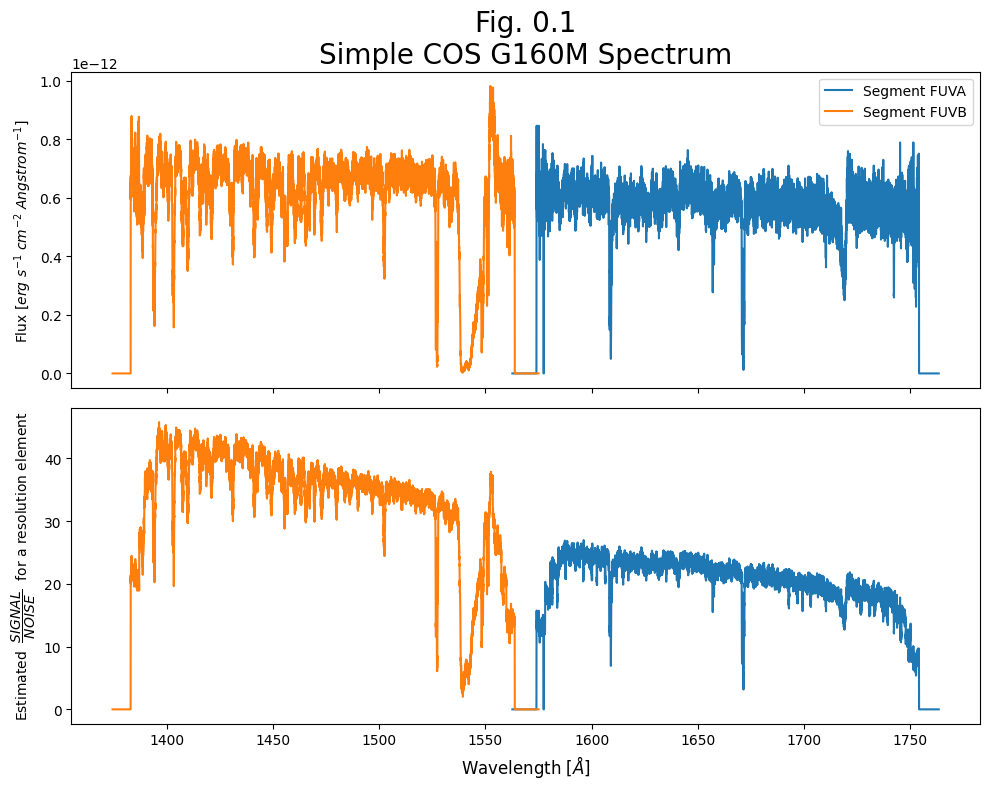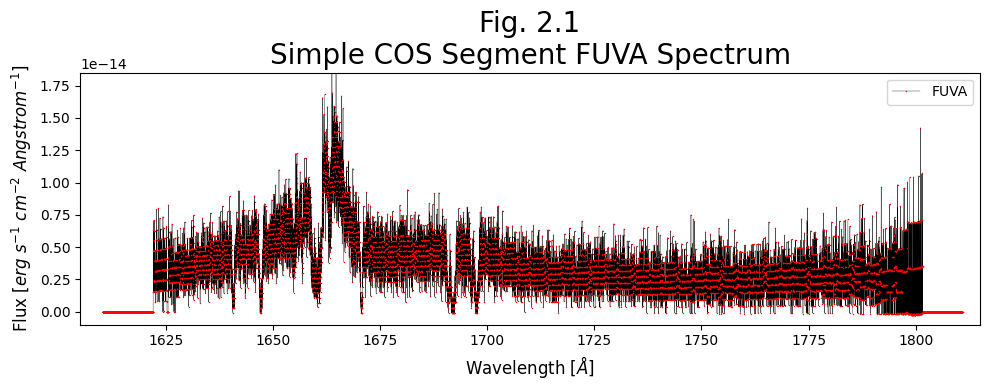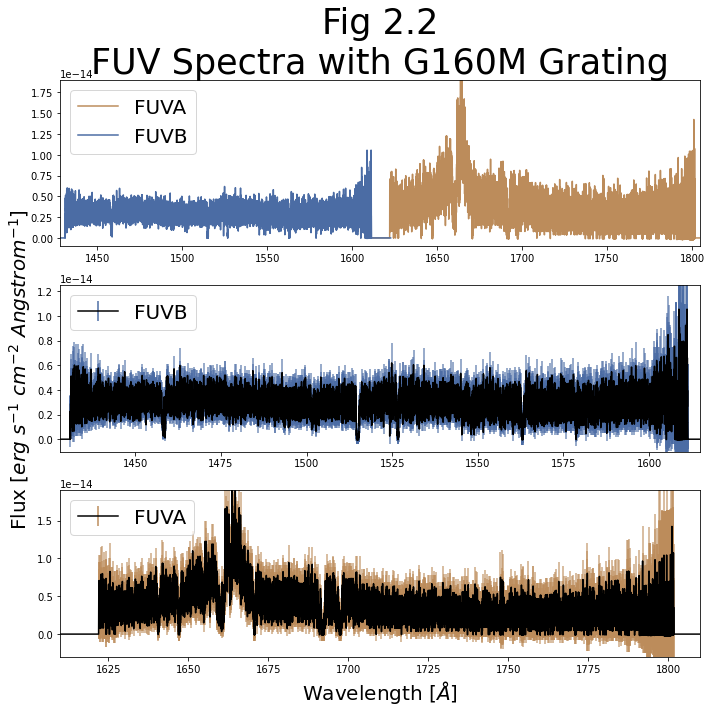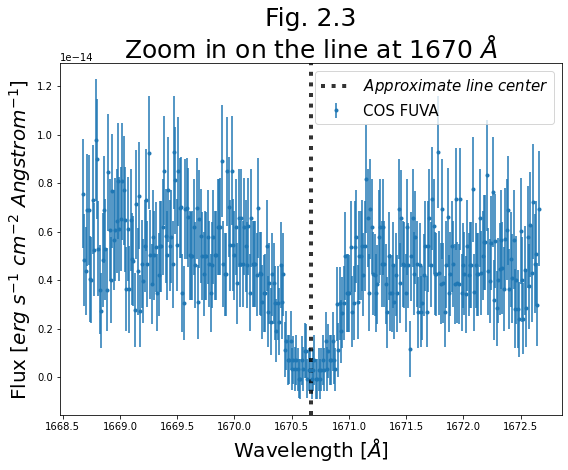# Learning Goals¶

This Notebook is designed to walk the user (you) through:

0. Introduction

1. Reading in the data using Python

2. Displaying the data using common plotting techniques

- 2.1. Plotting an FUV Spectrum

2.1.1. Our First Plot

- 2.2. Plotting an NUV Spectrum

3. Making a quick assessment of the data with tools to bin and measure the data's SNR and resolution

- 3.2. Binning the Data

3.2.2. Binning the FUV Data

3.3.3. Calculating the SNR

If you're confident with Reading-in and Plotting data in Python, you may run the first few cells defining directories and importing modules and then click the link when it appears to go ahead and skip to Section 3.

# 0. Introduction¶

The Cosmic Origins Spectrograph (COS) is an ultraviolet spectrograph on-board the Hubble Space Telescope (HST) with capabilities in the near ultraviolet (NUV) and far ultraviolet (FUV).

This tutorial aims to prepare you to begin analyzing COS data of your choice by walking you through reading and viewing a spectrum obtained with COS, as well as obtaining a few diagnostic measurements of your spectrum.

## 0.1. A one-cell summary of this Notebook's key points:¶

While the rest of this Notebook will walk you through each step and decision made when examining your COS data, the following code cell serves as a summary for the Notebook. It contains the key material condensed into a single code cell, without much explanation. If this is all the help you need, great! If you still have questions, read on!

In :
# This code cell condenses the key material of the Notebook into a single cell summary.

# 1. Import the necessary libraries:
from astropy.table import Table
from astropy.io import fits
from astroquery.mast import Observations
from cos_functions import estimate_snr
import matplotlib.pyplot as plt

Observations.filter_products(
Observations.get_product_list(
Observations.query_criteria(
)
),
productSubGroupDescription="X1DSUM"
)
)

# 3. Read in the data to an Astropy Table:
# Some users may be familiar with another way to read in FITS table data, though it can be less user-friendly:
with fits.open(onecell_x1dsum_data_products["Local Path"]) as hdulist:
alternate_x1dsum_data_structure = hdulist.data

# 4. Estimate the S/N ratio of the spectrum by calculating sqrt(counts):
#    The estimate_snr function is defined in the associated file: cos_functions.py
SNR_estimate = estimate_snr(x1dsum_data_table, binsize_=6, verbose=False)

# 5. Create a plot of the spectrum and the estimated S/N:
# Create 2-subplots and populate the upper one with the flux and the lower with S/N:
fig, (ax0, ax1) = plt.subplots(2, 1, figsize=(10, 8), sharex=True, dpi=100)
for i, segment_row in enumerate(x1dsum_data_table):
# In the upper panel, plot the spectrum as Flux over Wavelength:
ax0.plot(segment_row["WAVELENGTH"],
segment_row["FLUX"], label=f"Segment FUV{'AB'[i]}")
# In the lower panel, plot the S/N ratio as estimated S/N over Wavelength:
ax1.plot(SNR_estimate[i], SNR_estimate
[i], label=f"Segment FUV{'AB'[i]}")
# Format the plot and save
ax0.legend()
# Adds a title of fontsize 20 points
ax0.set_title("Fig. 0.1\nSimple COS G160M Spectrum", size=20)
# Adds y axis label to the top panel
ax0.set_ylabel('Flux [$erg\ s^{-1}\ cm^{-2}\ Angstrom^{-1}$]', size=10)
# Adds y axis label to the bottom panel
ax1.set_ylabel(
'Estimated  $\dfrac{SIGNAL}{NOISE}$  for a resolution element', size=10)
# Adds x axis label to the bottom panel
ax1.set_xlabel('Wavelength [$\AA$]', size=12)
plt.tight_layout()
plt.savefig("./Fig_0.1_quick_plot.png")

Downloading URL https://mast.stsci.edu/api/v0.1/Download/file?uri=mast:HST/product/ldm701020_x1dsum.fits to ./mastDownload/HST/ldm701020/ldm701020_x1dsum.fits ... [Done]

WARNING: UnitsWarning: 'erg /s /cm**2 /angstrom' contains multiple slashes, which is discouraged by the FITS standard [astropy.units.format.generic]• You will frequently see exclamation points (!) or dollar signs (\$) at the beginning of a line of code we are telling you to run. These are not part of the actual commands. The exclamation points tell a jupyter Notebook to pass the following line to the command line, and the dollar sign merely indicates the start of a terminal prompt. We will import the following packages: • numpy to handle array functions (version$\ge$1.17) • pathlib for managing system paths. • astropy.io fits for accessing FITS files • astropy.table Table for creating tidy tables of the data • astropy.units and astropy.visualization.quantity_support for dealing with units • matplotlib.pyplot for plotting data • astroquery.mast Observations for finding and downloading data from the MAST archive Later on, we will import some functions from a local file, cos_functions.py, which is installed as part of the same GitHub repository as this Notebook. If you do not see this Python file in this directory, you can find it here. cos_functions.py must be installed next to this Notebook (ViewData.ipynb). In : # Imports for: Downloading data from archive from astroquery.mast import Observations # Import for: Plotting import matplotlib.pyplot as plt # This line causes matplotlib plots to appear in the Notebook # instead of possibly showing up in separate windows %matplotlib inline # Import for: Manipulating arrays import numpy as np # Import for: Managing system variables and paths from pathlib import Path # Imports for: Reading in data from astropy.table import Table from astropy.io import fits # Imports for: Dealing with units and plotting them from astropy.visualization import quantity_support from astropy import units as u quantity_support()  Out: <astropy.visualization.units.quantity_support.<locals>.MplQuantityConverter at 0x7f9529ca5af0> We will also define a few directories in which to place our data and plots, as well as a few colors we will use in plots later. In : # These will be important directories for the Notebook datadir = Path('./data') outputsdir = Path('./output/') plotsdir = Path('./output/plots') # Make the directories if they don't exist datadir.mkdir(exist_ok=True), outputsdir.mkdir( exist_ok=True), plotsdir.mkdir(exist_ok=True) # Specifying a few arbitrary colors to correspond to COS segments for plotting by their hex code # Many search engines will show you the hex color - i.e. Google "#BC8C5B" to see this orange color segment_colors = {'FUVA': '#BC8C5B', 'FUVB': '#4B6CA4', 'NUVA': '#1813CE', 'NUVB': '#61946E', 'NUVC': '#8C1A11'}  We will be working with some FUV and NUV datasets downloaded in the cell below. These two datasets contain FUV observations of the QSO 3C48 and NUV observations of the Star WD1057 + 719, respectively. Searching for and downloading data is out of the scope of this tutorial. If you wish to learn more, please see our tutorial on downloading COS data. In : # Download the NUV data on WD1057+719 (with the G230L grating); only the _x1dsum and _asn nuv_downloads = Observations.download_products(Observations.get_product_list(Observations.query_criteria(obs_id='lbbd01020')), download_dir=str(datadir), extension='fits', mrp_only=True, cache=False) # Download the FUV data on QSO 3C48; only the _x1dsum and _asn fuv_downloads = Observations.download_products(Observations.get_product_list(Observations.query_criteria(obs_id='lcxv13050')), download_dir=str(datadir), extension='fits', mrp_only=True, cache=False)  Downloading URL https://mast.stsci.edu/api/v0.1/Download/file?uri=mast:HST/product/lbbd01020_asn.fits to data/mastDownload/HST/lbbd01020/lbbd01020_asn.fits ... [Done] Downloading URL https://mast.stsci.edu/api/v0.1/Download/file?uri=mast:HST/product/lbbd01020_x1dsum.fits to data/mastDownload/HST/lbbd01020/lbbd01020_x1dsum.fits ... [Done] Downloading URL https://mast.stsci.edu/api/v0.1/Download/file?uri=mast:HST/product/lcxv13050_asn.fits to data/mastDownload/HST/lcxv13050/lcxv13050_asn.fits ... [Done] Downloading URL https://mast.stsci.edu/api/v0.1/Download/file?uri=mast:HST/product/lcxv13050_x1dsum.fits to data/mastDownload/HST/lcxv13050/lcxv13050_x1dsum.fits ... [Done]  If you're confident Reading-in and Plotting data in Python, now go ahead to Section 3 # 1. Reading in the data¶ Calibrated COS 1-dimensional spectra are stored in fits files with the suffix x1d or x1dsum (1-dimensional here means that the cross-dispersion axis has been collapsed). x1d files contain a spectrum processed from a single exposure, while x1dsum files contain summed data from multiple exposures at different fixed pattern noise position settings (FP-POS's). You may also encounter x1dsumN files, i.e. x1dsum1/x1dsum2/x1dsum3/x1dsum4, which are intermediate files containing data from multiple exposures at the same FP-POS. All of these files are sub-types of x1d files and thus share the same basic data structure. What you learn with one filetype is transferable to working with another. We will be working with x1dsum files in this tutorial; however, please note that we often use the shorthand "x1d" as part of a variable name when programming, regardless of whether it is a summed file or not. The calibrated spectrum data has been downloaded onto our local machine as: <current-working-directory>/data/mastDownload/HST/<Obs_id>/<Obs_id>_x1dsum.fits, where the NUV and FUV Data are contained in the obs_ids: Spectral Region Obs_id Object Name Object Type filepath FUV LCXV13050 QSO 3C48 ./data/mastDownload/HST/lcxv13050 NUV LBBD01020 Star WD1057 + 719 ./data/mastDownload/HST/lbbd01020 ## 1.1. Investigating the Data - Basics¶ We want to learn the basics about this file, then read in the data. We can learn a great deal about our data from its primary fits header (see cell below). In : # Make sure these filepath variables point to your new FUV data # Note, We'll often refer to the x1dsum file with the prefix x1d for convenience # However, the files are x1dsum files, not the related x1d files - more info in the Data Handbook fuv_x1d_filepath = Path( './data/mastDownload/HST/lcxv13050/lcxv13050_x1dsum.fits') # This is the association file, used for processing spectra with CalCOS: fuv_asn_filepath = Path('./data/mastDownload/HST/lcxv13050/lcxv13050_asn.fits') # Make sure these point to your new NUV data nuv_x1d_filepath = Path( './data/mastDownload/HST/lbbd01020/lbbd01020_x1dsum.fits') # This is the NUV association file nuv_asn_filepath = Path('./data/mastDownload/HST/lbbd01020/lbbd01020_asn.fits') nuv_x1d_header = fits.getheader(nuv_x1d_filepath) nuv_asn_header = fits.getheader(nuv_asn_filepath) fuv_x1d_header = fits.getheader(fuv_x1d_filepath) fuv_asn_header = fits.getheader(fuv_asn_filepath) # This is the primary, 0th header of the calibrated nuv spectrum; fuv_x1d_header[:18], "...", fuv_x1d_header[45:50] # This is *not* the whole header... # ...The [:18] and [45:50] tell Python to print the first 18 lines, as well as lines 45-50  Out: (SIMPLE = T / conforms to FITS standard BITPIX = 8 / array data type NAXIS = 0 / number of array dimensions EXTEND = T NEXTEND = 1 / Number of standard extensions DATE = '2022-03-04' / date this file was written (yyyy-mm-dd) FILENAME= 'lcxv13050_x1dsum.fits' / name of file FILETYPE= 'SCI ' / type of data found in data file TELESCOP= 'HST' / telescope used to acquire data INSTRUME= 'COS ' / identifier for instrument used to acquire data EQUINOX = 2000.0 / equinox of celestial coord. system / DATA DESCRIPTION KEYWORDS ROOTNAME= 'lcxv13flq ' / rootname of the observation set IMAGETYP= 'TIME-TAG ' / type of exposure identifier PRIMESI = 'COS ' / instrument designated as prime , '...', / DIAGNOSTIC KEYWORDS PROCTIME= 5.964254436343E+04 / pipeline processing time (MJD) OPUS_VER= 'HSTDP 2021_5b ' / data processing software system versi AWSYSVER= ' ' / cloud infrastructure package version ) For instance, we notice that the FUV data was taken in TIME-TAG mode and calibrated with CalCOS version 3.3.10 (at the time of writing this sentence - it may have been reprocessed by the time you read this). However, some metadata information, such as the time of observation and calculated exposure time, can be found in the 1-th header rather than the 0th. We will read and print this below: In : with fits.open(fuv_x1d_filepath) as hdu: fuv_x1d_header1 = hdu.header fuv_date = fuv_x1d_header1['DATE-OBS'] fuv_time = fuv_x1d_header1['TIME-OBS'] fuv_exptime = fuv_x1d_header1['EXPTIME'] # It's also perfectly valid to access the 1-th extension header using 'fits.getheader(fuv_x1d_filepath, ext=1)' print( f"This FUV data was taken on {fuv_date} starting at {fuv_time} with a net exposure time of {fuv_exptime} seconds.") with fits.open(nuv_x1d_filepath) as hdu: nuv_x1d_header1 = hdu.header nuv_date = nuv_x1d_header1['DATE-OBS'] nuv_time = nuv_x1d_header1['TIME-OBS'] nuv_exptime = nuv_x1d_header1['EXPTIME'] print( f"This NUV data was taken on {nuv_date} starting at {nuv_time} with a net exposure time of {nuv_exptime} seconds.")  This FUV data was taken on 2016-06-13 starting at 23:56:29 with a net exposure time of 6532.512 seconds. This NUV data was taken on 2009-08-14 starting at 06:03:56 with a net exposure time of 999.136 seconds.  ## 1.2. Reading in the x1d/x1dsum Main Data¶ The data are in the 1-th header-data unit (HDU) of our fits file. There are several ways to read in our data from the our table-formatted fits file. We'll demonstrate three common methods below, focusing in on the astropy.table method. We will then display all the fields contained in this data table using the .colnames method. You can ignore the warnings about multiple slashes in the units that come up while reading in the data. The proper units are displayed in LaTex as: • 'erg /s /cm**2 /angstrom' ==> $$\ \ erg\ s^{-1}\ cm^{-2}\ \mathring{A}^{-1}$$ • 'count /s /pixel' ==> $$\ \ counts\ s^{-1}\ pixel^{-1}$$ The columns of these tables include some scalar values which describe the data (i.e. EXPTIME), while the columns containing actual data hold it in arrays of equal length (i.e. WAVELENGTH, FLUX, etc., where that length = NELEM). In : # Method number 1: with fits.open(fuv_x1d_filepath) as hdulist: fuv_x1d_data = hdulist.data print(f"Method 1 gets data as type: {type(fuv_x1d_data)}") # Method number 2: fuv_x1d_data = fits.getdata(fuv_x1d_filepath) print(f"Method 2 gets data as type: {type(fuv_x1d_data)}") # Method number 3: fuv_x1d_data = Table.read(fuv_x1d_filepath) print(f"Method 3 gets data as type: {type(fuv_x1d_data)}") # We can easily explore this astropy table columns = fuv_x1d_data.colnames # Print basic info about the table's columns print("\nTable of FUV data with columns:\n", columns, "\n") # Display a representation of the data table: fuv_x1d_data  Method 1 gets data as type: <class 'astropy.io.fits.fitsrec.FITS_rec'> Method 2 gets data as type: <class 'astropy.io.fits.fitsrec.FITS_rec'>  WARNING: UnitsWarning: 'erg /s /cm**2 /angstrom' contains multiple slashes, which is discouraged by the FITS standard [astropy.units.format.generic]  Method 3 gets data as type: <class 'astropy.table.table.Table'> Table of FUV data with columns: ['SEGMENT', 'EXPTIME', 'NELEM', 'WAVELENGTH', 'FLUX', 'ERROR', 'ERROR_LOWER', 'GROSS', 'GCOUNTS', 'VARIANCE_FLAT', 'VARIANCE_COUNTS', 'VARIANCE_BKG', 'NET', 'BACKGROUND', 'DQ', 'DQ_WGT']  Out: Table length=2 SEGMENTEXPTIMENELEMWAVELENGTH FLUX ERROR ERROR_LOWER GROSS GCOUNTS VARIANCE_FLAT VARIANCE_COUNTS VARIANCE_BKG NET BACKGROUND DQ DQ_WGT  sAngstromerg / (Angstrom cm2 s)erg / (Angstrom cm2 s)ct / sct / sctctctctct / sct / s bytes4float64int32float64float32float32float32float32float32float32float32float32float32float32int16float32 FUVA6532.512163841610.2408223563473 .. 1810.94485265342970.0 .. 0.00.0 .. 0.00.0 .. 0.00.0 .. 0.00.0 .. 0.00.0 .. 0.00.0 .. 0.00.0 .. 0.00.0 .. 0.00.0 .. 0.0128 .. 1280.0 .. 0.0 FUVB6532.512163841421.946456029049 .. 1622.59581877655820.0 .. 0.00.0 .. 0.00.0 .. 0.00.0 .. 0.00.0 .. 0.00.0 .. 0.00.0 .. 0.00.0 .. 0.00.0 .. 0.00.0 .. 0.0128 .. 1280.0 .. 0.0 In the case of the FUV data, we see an astropy style table of 2 rows which are labeled FUVA and FUVB. These rows contain data from the 2 segments of FUV Detector (see figure 1.1). ### Fig. 1.1 from COS DHB Fig. 1.6¶In the case of the NUV data, we see a similar astropy style table of 3 rows (labeled NUVA, NUVB, and NUVC). These rows contain data from the 3 stripes of the NUV spectrum (see figure 1.2). ### Fig. 1.2 from COS DHB Fig. 1.10¶An important thing to note about this NUV data in particular is that with the grating used here (G230L), stripe C is actually a 2nd order spectrum with a higher dispersion and ~5% contamination from the 1st order spectrum. See the COS Data Handbook, especially Fig. 1.3, for more information. ## 1.3. The Association (asn) file¶ It's also likely we will want to see what observations went into making this calibrated spectrum. This information is contained in the Association (asn) file, under the MEMNAME column. In : print(fits.info(fuv_asn_filepath), '\n\n----\n') fuv_asn_data = Table.read(fuv_asn_filepath) print(fuv_asn_data)  Filename: data/mastDownload/HST/lcxv13050/lcxv13050_asn.fits No. Name Ver Type Cards Dimensions Format 0 PRIMARY 1 PrimaryHDU 43 () 1 ASN 1 BinTableHDU 25 5R x 3C [14A, 14A, L] None ---- MEMNAME MEMTYPE MEMPRSNT -------------- -------------- -------- LCXV13FLQ EXP-FP 1 LCXV13FXQ EXP-FP 1 LCXV13G4Q EXP-FP 1 LCXV13GXQ EXP-FP 1 LCXV13050 PROD-FP 1  We see that our data has MEMTYPE = PROD-FP, meaning it is an output science product (see COS DHB Table 2.6.) This particular association file lists four EXP-FP (input science exposure), with the MEMNAME values (Dataset IDs) [LCXV13FLQ, LCXV13FXQ, LCXV13G4Q, LCXV13GXQ]. We could look for these datasets, if we wished to inspect the exposures individually. ### Exercise 1.1. Finding Metadata for the NUV¶ 1. Read in the NUV data, just as we did with the FUV data. 2. From the 0th header of the x1dsum file, determine the time (in MJDs) that the data was processed. (keyword = PROCTIME) and from the 1th header, determine how many wavelength calibration "flashes" were used (keyword = NUMFLASH) 3. From the asn file, determine how many input science exposures went into the NUV x1dsum file. In : # Your code here  # 2. Plotting the Data¶ ## 2.1. Plotting an FUV Spectrum¶ Let's select the simplest data we need to plot a spectrum: WAVELENGTH, FLUX, and ERROR. • Note that here, ERROR is flux error, which is discussed in the COS Instrument Handbook • Also note that somewhat counterintuitively, the FUVB segment extends over shorter wavelengths than the FUVA. ### 2.1.1. Our First Plot¶ We begin with a simple plot: simply a line plot of a single segment (FUVA) without its error. The goal of this show is to demonstrate some of the common parameters of making a simple plot with matplotlib which you are likely to often need. The comments explain what each line does. In : #  Access FUVA data, the longer wvln segment and gets the data we need to plot a spectrum: wvln, flux, segment = fuv_x1d_data["WAVELENGTH", "FLUX", "SEGMENT"] # Set up the plot as a single box with size of 10x4 inches, and with a dpi of 100, relevant should we choose to save it: fig1, ax = plt.subplots(1, 1, figsize=(10, 4), dpi=100) # The next few lines are the core of the cell, where we actually place the data onto the plot: ############### ax.plot(wvln, flux, # First two arguments are assumed to be the x-data, y-data # These parameters specify the look of the connecting line linestyle="-", linewidth=0.25, c='black', # The marker parameters specify how the data points will look... marker='.', markersize=2, markerfacecolor='r', markeredgewidth=0, # ... if you don't want dots set marker='' label=segment) # The label is an optional parameter which will allow us to create a legend # this label is useful when there are multiple datasets on the same plot # The lines after this are all about formatting, adding text, and saving as an image ############### ax.set_title("Fig. 2.1\nSimple COS Segment FUVA Spectrum", size=20) # Adds a title of fontsize 20 points ax.set_xlabel('Wavelength [$\AA$]', size=12) # Adds x axis label # Adds y label ax.set_ylabel('Flux [$erg\ s^{-1}\ cm^{-2}\ Angstrom^{-1}]', size=12) # These two lines set the x and y bounds of the image in whatever units we are plotting in plt.xlim(1605, 1815) plt.ylim(-1E-15, 1.85E-14) # Adds a legend with the label specified in the plotting call plt.legend(loc='upper right') plt.tight_layout() # Trims blank space # Optionally you can save the plot as an image plt.savefig(str(plotsdir / "Fig2.1.png")) plt.show() # Shows all the plot calls in this cell and "clears" the plotting space. Must come after any saving you want to do### Exercise 2.1: A clearer plot¶ Plot the data from Segment FUVB, similar to the Segment FUVA plot above, but this time, normalize flux to a max of 1, and don't plot the red markers, which can be distracting. Note what can you simply copy over, and what you have to make sure to change. In : # Your code here  ### 2.1.2. A Complex Look at the Entire FUV¶ Now that we have an idea for how matplotlib works, let's make a more complicated graph showing both FUV segments - independently and together. One of the most important steps to creating a plot is planning out how it will look and convey its information. We'll begin by planning this out below: Panel Contents Information Conveyed Notes top Entire FUV Spectrum as a simple plot Overview of the entire spectrum we have, coarse look without much detail Color by segment middle Shorter Wavelength FUVB Spectrum as an errorbar plot Closer look at the shorter wavelength spectrum with an idea of error Color errorbar by segment, central line in black bottom Longer Wavelength FUVA Spectrum as an errorbar plot Closer look at the longer wavelength spectrum with an idea of error Color errorbar by segment, central line in black In : # ax0, ax1, ax2 are our 3 vertically-aligned panels: top, middle, and bottom fig, (ax0, ax1, ax2) = plt.subplots(3, 1, figsize=(10, 10)) # Repeats for i=[0,1] to apply to each segment's data at a time for i in range(2): # Selects all useful data for the chosen segment wvln, flux, fluxErr, segment = fuv_x1d_data[i]["WAVELENGTH", "FLUX", "ERROR", "SEGMENT"] # This section applies the top (0th) panel's plotting and formatting: ax0.plot(wvln, flux, linestyle="-", label=segment, c=segment_colors[segment]) ax0.legend(fontsize=20, loc='upper left') ax0.set_title("Fig 2.2\nFUV Spectra with G160M Grating", size=35) ax0.set_xlim(1428, 1805) ax0.set_ylim(-1E-15, 1.9E-14) ###### if i == 0: # This indented code applies only to segment FUVA data in bottom Panel markers, caps, bars = ax2.errorbar(x=wvln, y=flux, yerr=fluxErr, linestyle="-", label=segment, marker='', markersize=1, c='k', alpha=1, ecolor=segment_colors[segment]) ax2.set_xlim(1610, 1810) ax2.set_ylim(-3E-15, 1.9E-14) ax2.legend(fontsize=20, loc='upper left') ax2.set_xlabel('Wavelength [\AA$]', size=20) [bar.set_alpha(0.75) for bar in bars] ###### if i == 1: # This indented code applies only to segment FUVB data in middle Panel markers, caps, bars = ax1.errorbar(x=wvln, y=flux, yerr=fluxErr, linestyle="-", label=segment, c='k', ecolor=segment_colors[segment]) ax1.set_xlim(1428, 1615) ax1.set_ylim(-1E-15, 1.25E-14) ax1.legend(fontsize=20, loc='upper left') ax1.set_ylabel('Flux [$erg\ s^{-1}\ cm^{-2}\ Angstrom^{-1}$]', size=20) [bar.set_alpha(0.75) for bar in bars] ###### plt.tight_layout() plt.savefig(str(plotsdir / 'Fig2.2.png'), dpi=200) plt.show()### 2.1.3. Looking Closer at Parts of the FUV Spectrum¶ It can be very difficult to get any insights on small-scale details in the above plots because data is too dense to parse at once. Below, we'll show examples of: • Plotting a small region around an absorption line feature. • Plotting an entire segment's spectrum in segments of wavelength space. Let's begin with showing a region around a line, in this case, we see a sharp line around 1670 Å. In : line1670 = 1670.67 # By-eye center of the line for plotting # Selects all useful data for the chosen segment wvln, flux, fluxErr, segment = fuv_x1d_data["WAVELENGTH", "FLUX", "ERROR", "SEGMENT"] wvln_extent = 2 # How many Angstroms in each direction around line1670 do we want to look at? # Mask the data to within +/- wvln_extent Angstrom of the line - speeds up the plotting lineRegion_mask = (wvln > line1670 - wvln_extent) & (wvln < line1670 + wvln_extent) # applies the created mask wvln_region, flux_region, fluxErr_region = wvln[ lineRegion_mask], flux[lineRegion_mask], fluxErr[lineRegion_mask] plt.figure(figsize=(8, 6)) # how big should the plot be plt.axvline(x=line1670, c='k', linewidth=4, linestyle='dotted', # vertical line at x position line1670; color = 'k' AKA black, line thickness = 4 points, type of line is dotted alpha=0.8, label="$Approximate\ line\ center$") # alpha sets transparency where 1 is opaque; label for the legend plt.errorbar(x=wvln_region, y=flux_region, yerr=fluxErr_region, label='COS FUVA', linestyle='', marker='.') # marker tells the point to look like a dot plt.xlabel('Wavelength [$\AA$]', size=20) plt.ylabel('Flux [$erg\ s^{-1}\ cm^{-2}\ Angstrom^{-1}$]', size=20) plt.legend(fontsize=15) plt.tight_layout() plt.savefig(str(plotsdir / 'Fig2.3.png'), dpi=200) plt.title("Fig. 2.3\nZoom in on the line at 1670$\AA$", size=25)  Out: Text(0.5, 1.0, 'Fig. 2.3\nZoom in on the line at 1670$\\AA$')The source we're looking at - the quasar 3C48 has a substantial redshift: $$z \approx 0.37$$ Source: Simbad search for 3C48 With that redshift, we can determine the rest-frame wavelength. $$z = \dfrac{\lambda_{obs}-\lambda_{rest}}{\lambda_{rest}} \ \ \rightarrow\ \ \ \lambda_{rest} = \dfrac{\lambda_{obs}}{1+z}$$ At that redshift, we see this line to be$\approx 1219$Å. This is close to, and may correspond to, the Lyman-$\alpha$transition. Now we will create a much more complex plot, allowing us to visualize an entire segment's spectrum in fine detail We'll split the spectrum into "segments" of ~10 Angstroms, and plot these segments in a vertical series. These plots may take a minute to create. If you want them to run quicker, hit the Interrupt the Kernel square-shaped button to stop the current cell from running (you will get an error message), and run this cell with a smaller number of rows. In : %%time # Let's see how long it takes with the above "Cell magic" ... # (Cell magic is a Jupyter/iPython series of utilities: https://ipython.readthedocs.io/en/stable/interactive/magics.html) for segment_row in fuv_x1d_data: # Apply the following to each segment's data at a time # Selects all immediately useful data for the chosen segment wvln, flux, fluxErr, segment = segment_row["WAVELENGTH", "FLUX", "ERROR", "SEGMENT"] minx, maxx = min(wvln), max(wvln) miny, maxy = min(flux), max(flux) rangex = maxx - minx fig = plt.figure(figsize=(14, 20)) nRows = 15 # How many segments we wish to split the spectrum into for i in range(nRows): min_ = minx + i*rangex/nRows max_ = minx + (i+1)*rangex/nRows ax = plt.subplot(nRows, 1, i+1) if i == 0: # A way to set Title, xlabel, and ylabel that will work independent of number of rows ax.set_title( f"Fig. 2.4{segment[-1]} \nSegment {segment} Spectrum split into segments", size=30) if i == nRows - 1: ax.set_xlabel("Wavelength [$\AA$]", size=30) if i == int(nRows/2): ax.set_ylabel( 'Flux [$erg\ s^{-1}\ cm^{-2}\ Angstrom^{-1}\$]', size=30)

# Create the plot itself
ax.errorbar(wvln, flux, fluxErr, c=plt.cm.rainbow((i+1)/nRows), alpha=0.8,
marker='.', markerfacecolor='k', markersize=2, mew=0)

ax.set_xlim(min_, max_)
plt.tight_layout()
plt.savefig(
str(plotsdir / f'Fig2.4{segment[-1]}_{nRows}Rows_seg{segment}.png'), dpi=200)
plt.show()
print("\n----\n")